www.dlr.de · Antonin RAFFIN · RL Tips and Tricks · RLVS · 09.04.2021

### RL Tips and Tricks

and The Challenges of Applying RL to Real Robots

Antonin RAFFIN (@araffin2)
German Aerospace Center (DLR)
https://araffin.github.io/

### What is this session about?

• Part I: RL Tips and Tricks and Examples on Real Robots
• Part II: Hands-on Session with Stable-Baselines3 (SB3)

### Outline

1. RL Tips and Tricks
1. General Nuts and Bolts of RL Experimentation
2. RL in practice on a custom task
3. Questions?
2. The Challenges of Applying RL to Real Robots
1. Learning to control an elastic robot
2. Learning to drive in minutes and learning to race in hours
3. Learning to walk with an elastic quadruped robot
4. Questions?

## RL Tips And Tricks

### RL is Hard (1/2)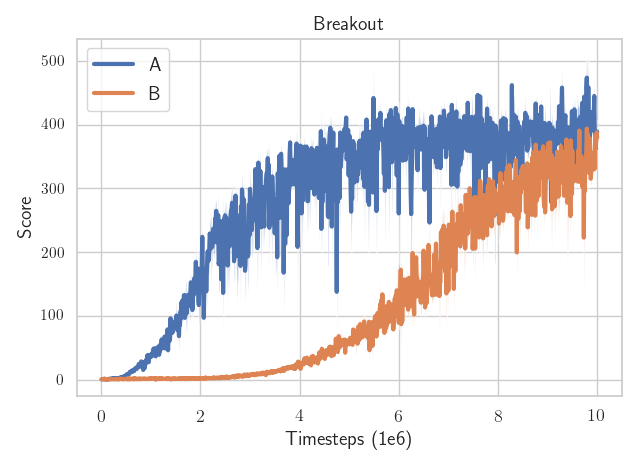Which algorithm is better?

The only difference: the epsilon value to avoid division by zero in the optimizer (one is eps=1e-7 the other eps=1e-5)

### RL is Hard (2/2)

• data collection by the agent itself
• sensitivity to the random seed / hyperparameters
• sample inefficient
• reward function design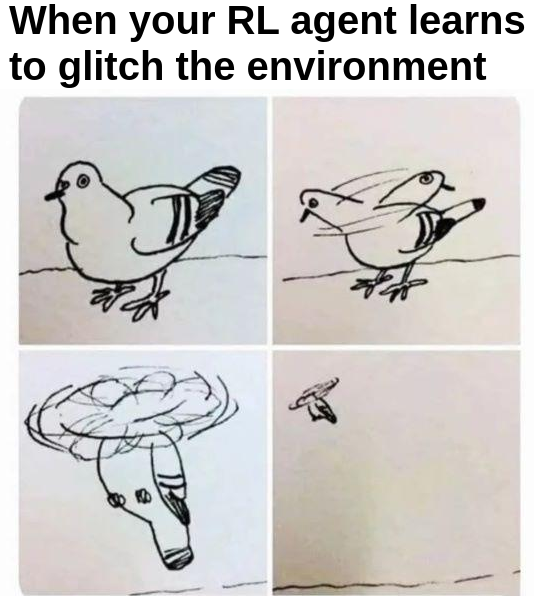Credits: Rishabh Mehrotra (@erishabh)

### Best Practices

• quantitative evaluation
• use recommended hyperparameters
• save all experiments parameters
• use the RL zoo

### Do you really need RL?• observation space
• action space
• reward function
• termination conditions

### Choosing the observation space

• enough information to solve the task
• do not break Markov assumption
• normalize!

### Choosing the Action space

• discrete / continuous
• complexity vs final performance

### Continuous action space: Normalize? Normalize!


from gym import spaces

# Unnormalized action spaces only work with algorithms
# that don't directly rely on a Gaussian distribution to define the policy
# (e.g. DDPG or SAC, where their output is rescaled to fit the action space limits)

# LIMITS TOO BIG: in that case, the sampled actions will only have values
# around zero, far away from the limits of the space
action_space = spaces.Box(low=-1000, high=1000, shape=(n_actions,), dtype="float32")

# LIMITS TOO SMALL: in that case, the sampled actions will almost
# always saturate (be greater than the limits)
action_space = spaces.Box(low=-0.02, high=0.02, shape=(n_actions,), dtype="float32")

# BEST PRACTICE: action space is normalized, symmetric
# and has an interval range of two,
# which is usually the same magnitude as the initial standard deviation
# of the Gaussian used to sample actions (unit initial std in SB3)
action_space = spaces.Box(low=-1, high=1, shape=(n_actions,), dtype="float32")### Choosing the reward function

• primary / secondary reward
• normalize!

### Termination conditions?

• early stopping
• special treatment needed for timeouts
• should not change the task (reward hacking)

### Which algorithm to choose?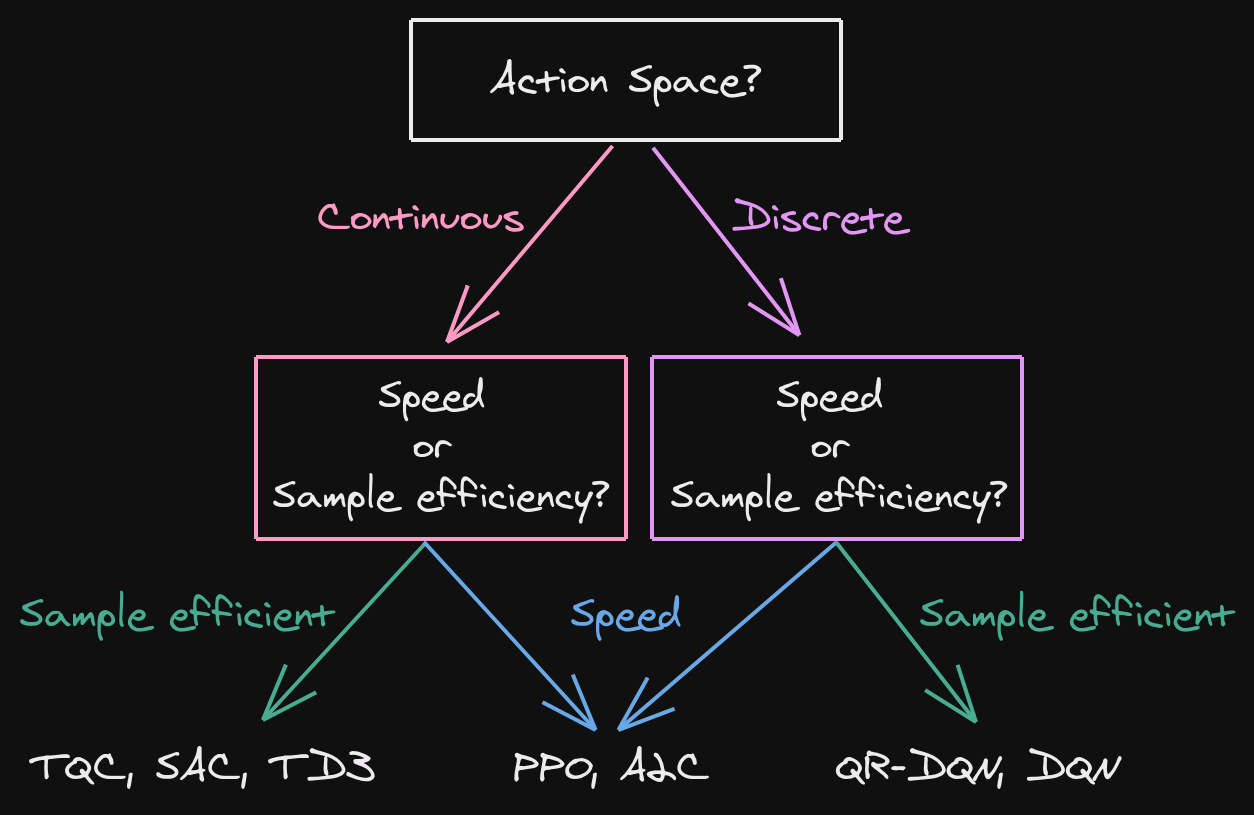### It doesn't work!

• did you follow the best practices?
• start simple
• use trusted implementations
• increase budget
• hyperparameter tuning (Optuna)

#### Recap

• RL is hard
• do you need RL?
• best practices

## 2. The Challenges of Applying RL to Real Robots

### Simulation is all you need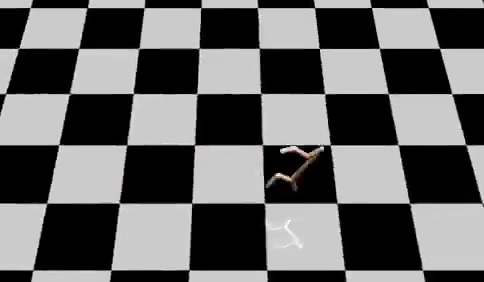Credits: Nathan Lambert (@natolambert)

### Why learn directly on real robots?

• simulation is safer, faster
• simulation to reality (sim2real): accurate model and randomization needed
• challenges: robot safety, sample efficiency

#### Learning to control an elastic robot

##### Challenges
• hard to model (silicon neck)
• oscillations
• real robot (safety)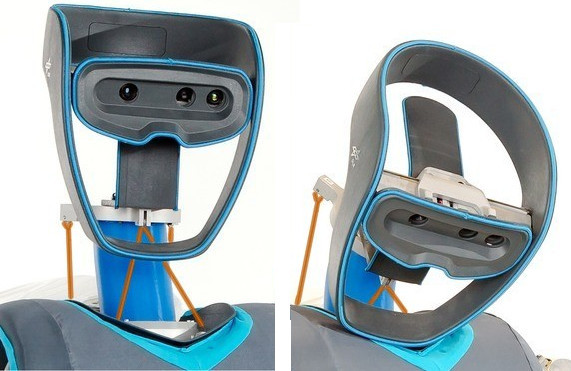#### Generalized State-Dependent Exploration (gSDE)

Independent Gaussian noise: $\epsilon_t \sim \mathcal{N}(0, \sigma)$ $a_t = \mu(s_t; \theta_{\mu}) + \epsilon_t$
State dependent exploration: $\theta_{\epsilon} \sim \mathcal{N}(0, \sigma_{\epsilon})$ $a_t = \mu(s_t; \theta_{\mu}) + \epsilon(s_t; \theta_{\epsilon})$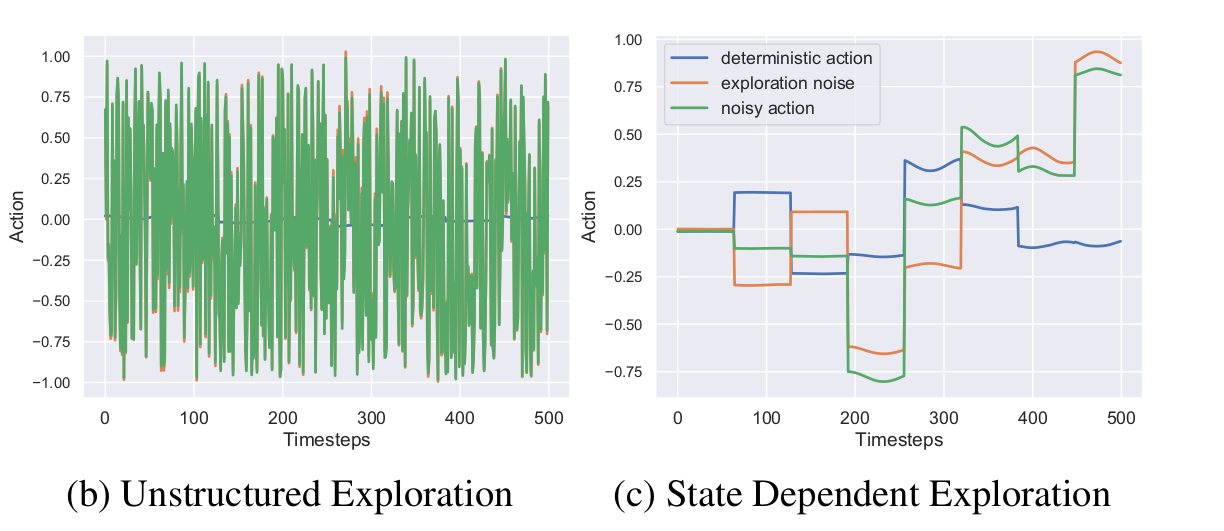#### Continuity Cost

• formulation: $r_{continuity} = - (a_t - a_{t - 1})^2$
• requires a history wrapper
• can be done in the loss function

ObservationSpace tendon forces, desired pose, current pose desired forces (4D) distance to target / continuity success / timeout SAC + gSDE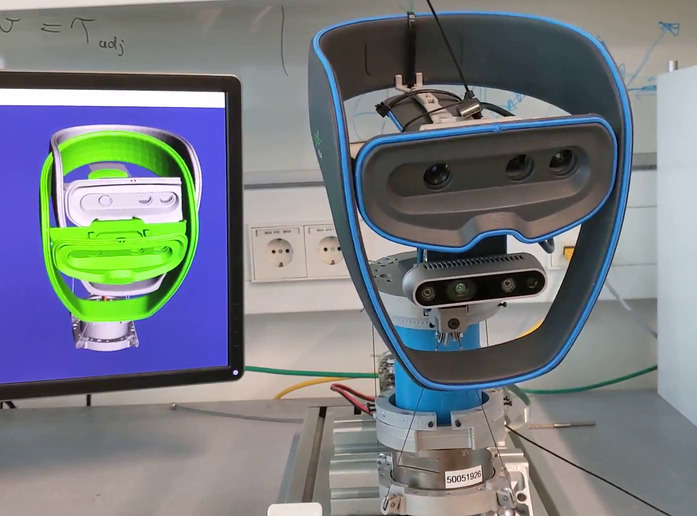#### Results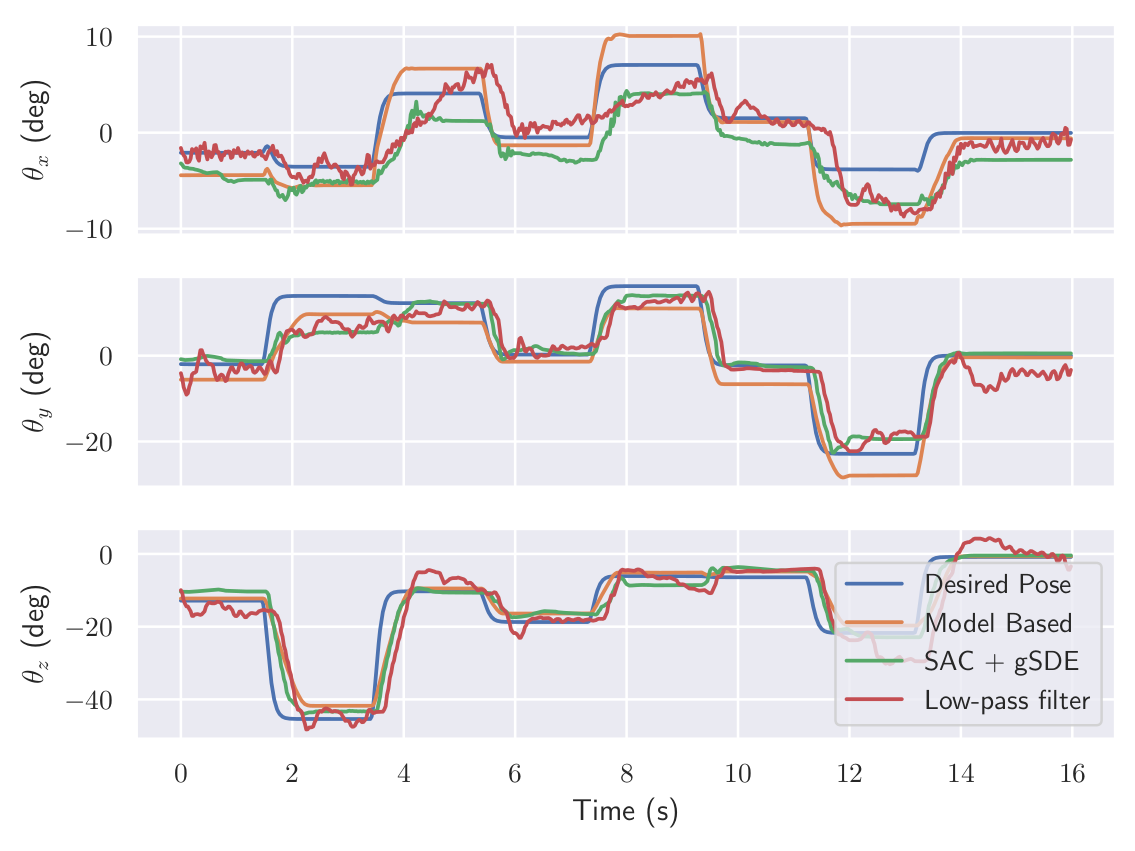#### Learning to drive in minutes / learning to race in hours

##### Challenges
• minimal number of sensors (image, speed)
• variability of the scene (light, shadows, other cars, ...)
• oscillations
• limited computing power
• communication delay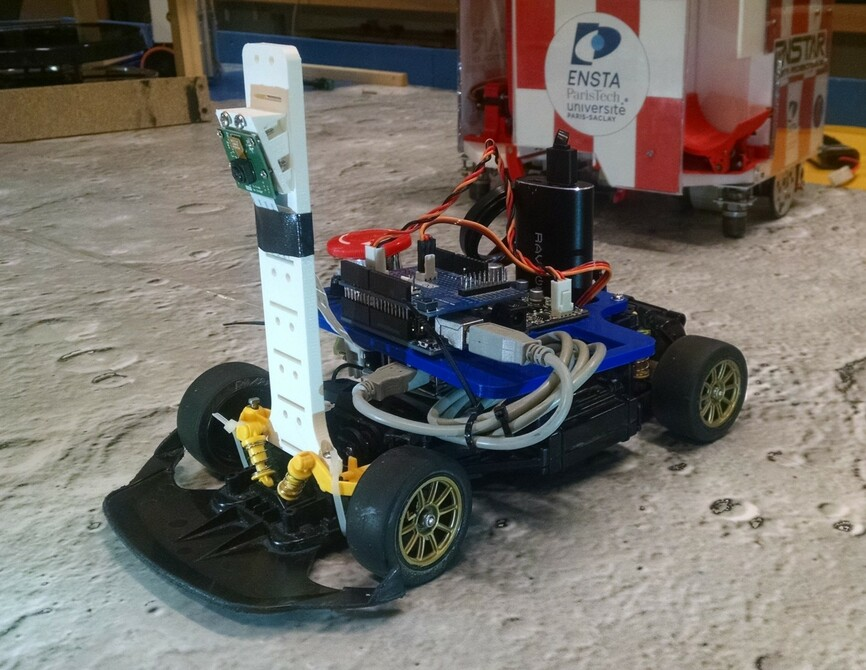#### Learning a state representation (SRL)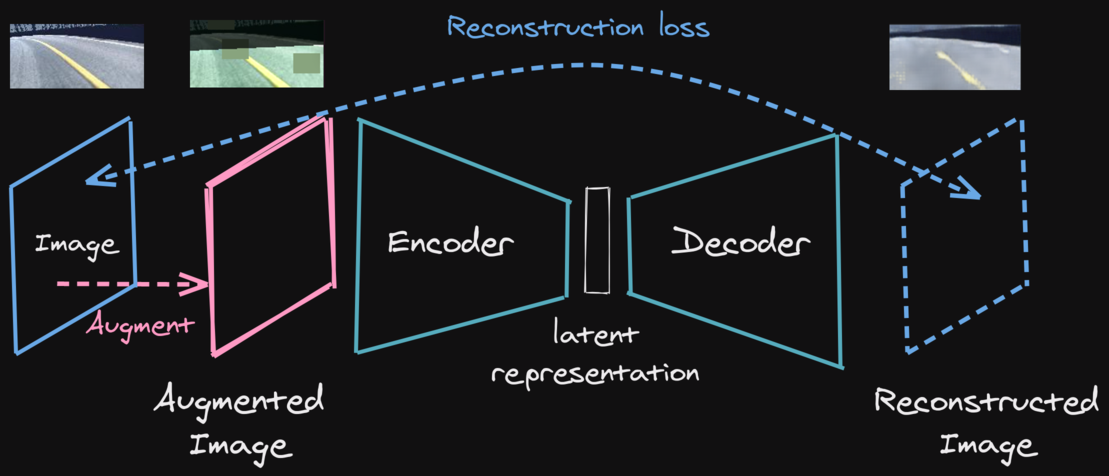ObservationSpace latent vector / current speed + history steering angle / throttle speed + smoothness crash / timeout SAC / TQC + gSDE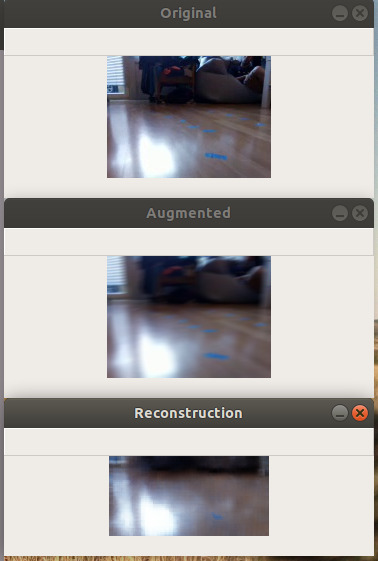#### Learning to walk with an elastic quadruped robot

##### Challenges
• hardcoded solution possible (CPG) but need tuning / not energy efficient / fast
• robot safety
• manual reset
• communication delay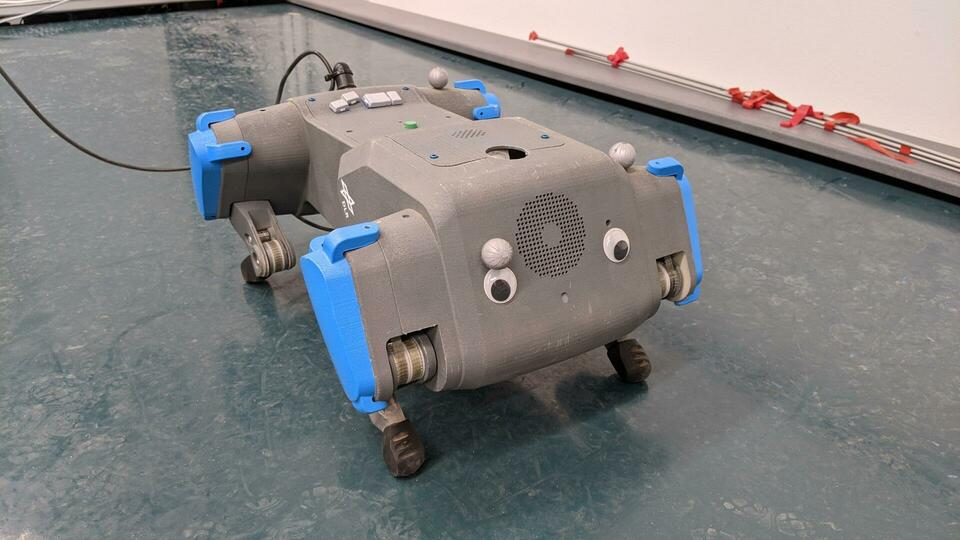ObservationSpace joints positons / torques / imu / gyro + history motor positions (6D) forward distance / walk straight / continuity fall / timeout TQC + gSDE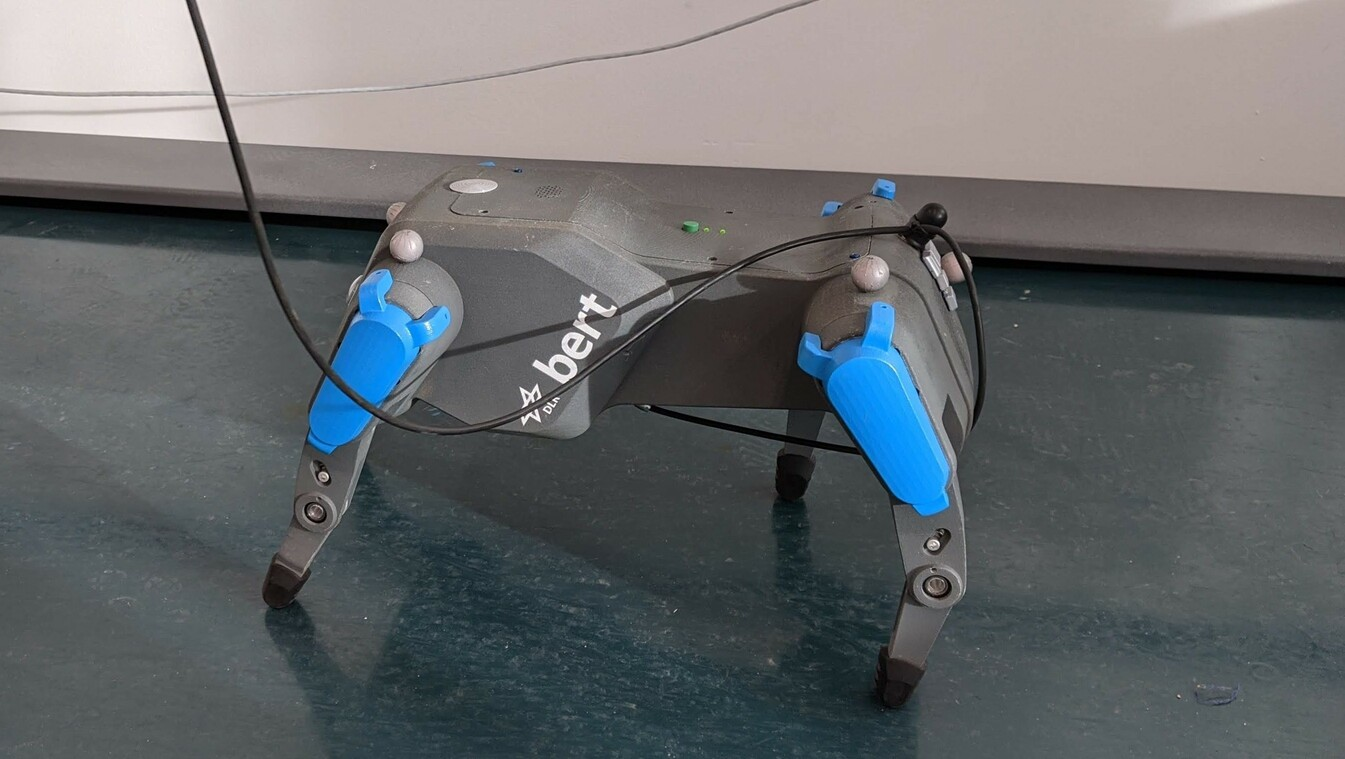#### Recap

• simulation is all you need
• learning directly on a real robot
• smooth control
• decoupling features extraction from policy learning

### Coming Next: Hands-on Session with Stable Baselines3

Notebook repo: https://github.com/araffin/rl-handson-rlvs21

### Backup slides

#### Who am I?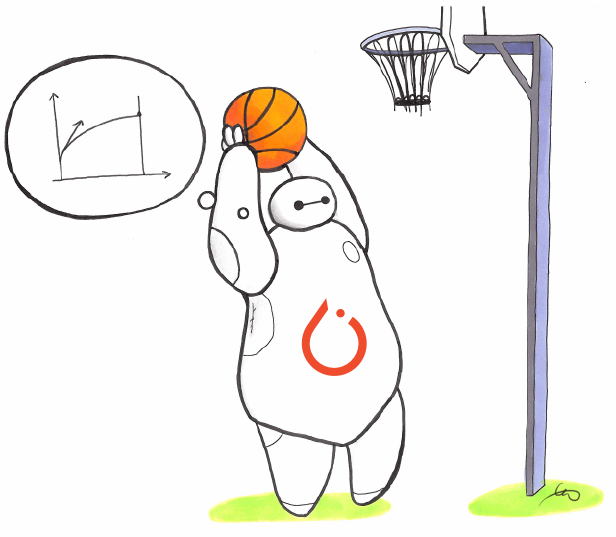Stable-Baselines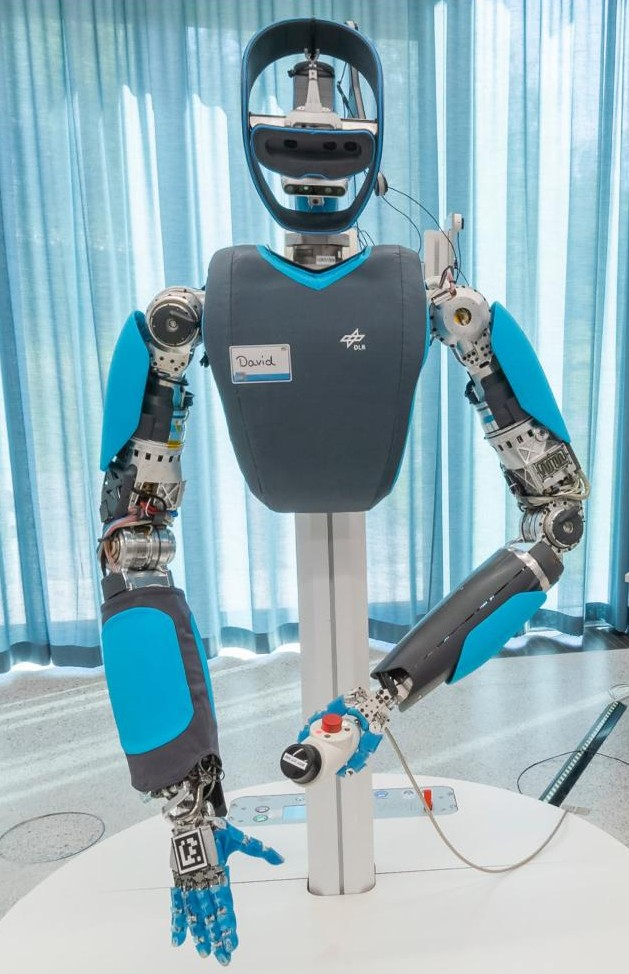David (aka HASy)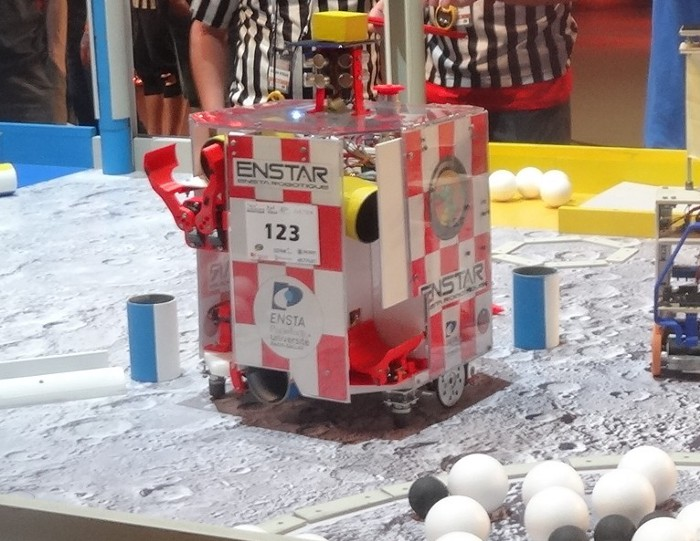ENSTA Robotique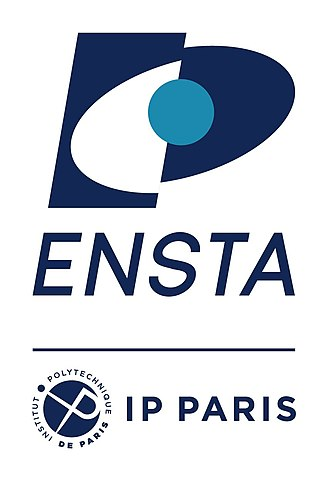ENSTA Paris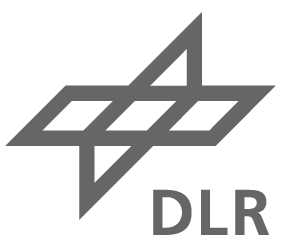German Aerospace Center (DLR)